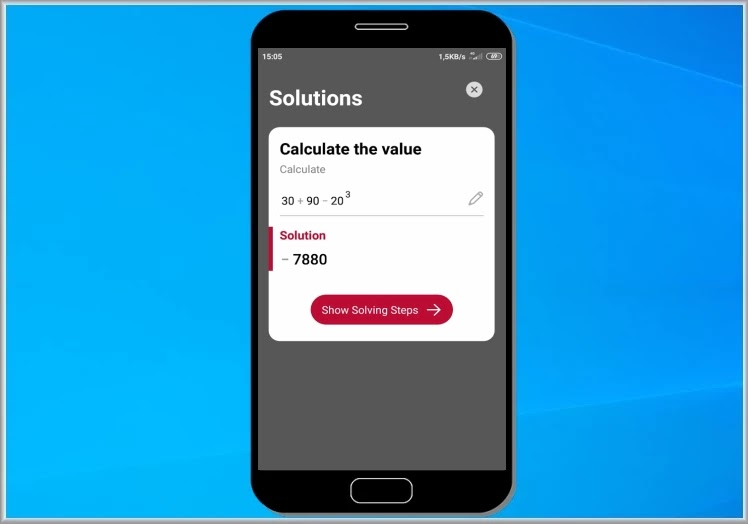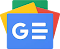## Thursday April 21 2022In an earlier post we talked about it symbolab, an online application with the help of which you can solve the most difficult mathematical problems. In the opposite of this application we present PhotoMath, an application for mobile phones that undertakes on your behalf to solve the most difficult problems. Its strong point is that we do not need to type anything and all we have to do is focus with the camera of our mobile on the mathematical operation that we have to perform and automatically the application will give us the solution. It can solve operations of simple arithmetic, integers, decimal fractions, roots, factors, linear equations, inequalities, quadratic equations, equation systems, logarithms, functions, arrays, graphs, polynomials and anything you can imagine with a simple scan. The method is very simple, write in a notebook the operation you want to solve and scan it with the camera of your mobile, on the next screen the solution will appear immediately.Follow us on Google News